# Löwner equation

(diff) ← Older revision | Latest revision (diff) | Newer revision → (diff)

A differential equation of the form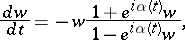where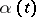is a real-valued continuous function on the interval. A generalization of the Löwner equation is the Kufarev–Löwner equation:where,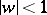,, is a function measurable infor fixedand regular in, with positive real part, normalized by the condition. The Löwner equation and the Kufarev–Löwner equation, which arise in the theory of univalent functions, are the basis of the variation-parametric method of investigating extremal problems on conformal mapping.

The solution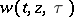,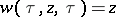, of the Kufarev–Löwner equation, regarded as a function of the initial value, for any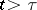maps the disc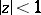conformally onto a one-sheeted simply-connected domain belonging to the disc. From the formula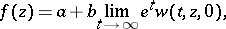by a suitable choice of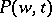in the Kufarev–Löwner equation and complex constants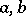one can obtain an arbitrary regular univalent function in the disc. In this way the Löwner equation generates, in particular, the conformal mappings of the disc onto domains obtained from the whole plane by making a slit along some Jordan arc (see ).

The partial differential equation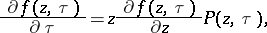which is satisfied by the functionis also called the Kufarev–Löwner equation.

The Löwner equation was set up by K. Löwner ; the Kufarev–Löwner equation was obtained by P.P. Kufarev (see ).

How to Cite This Entry:
Löwner equation. Encyclopedia of Mathematics. URL: http://encyclopediaofmath.org/index.php?title=L%C3%B6wner_equation&oldid=12765
This article was adapted from an original article by V.Ya. Gutlyanskii (originator), which appeared in Encyclopedia of Mathematics - ISBN 1402006098. See original article